# Abstract

## 1. Introduction

Curriculum Learning （CL）受人类和动物认知过程的启发，找到了一种解决以上问题的方法，那就是对训练样本进行一个排序，更多地关注最有可能是正确的样本。

• 对于带有受污染数据（损坏标签）的deep CNNs作者提出了一种学习数据驱动课程的新方法。
• 讨论了一种利用小批量随机梯度下降法进行深度网络课程学习的算法。
• 在4个基准测试上验证了我们的方法，并在WebVision基准测试上获得了目前发布的最好的结果。

# 2. Preliminary on Curriculum Learning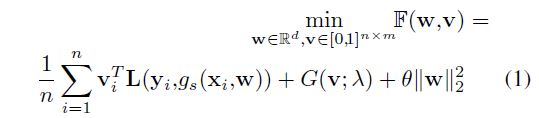${g}_{s}$$g_s$中包含了data augmentation和dropout。${v}_{i}\in \left[0,1{\right]}^{m×1}$$v_i \in [0,1]^{m \times 1}$是一个表示第$i$$i$个样本潜在权重变量的向量。$G$$G$就是代表课程。这篇文章使用one-hot编码，为了表示方便,使用${l}_{i}$$l_i$表示损失，标量${v}_{i}$$v_i$表示潜在权重变量，整数${y}_{i}\in \left[1,m\right]$$y_i \in [1,m]$标签。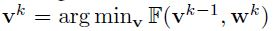$v$$v$进行更新。例如，可令$G\left(v\right)=-\lambda {‖v‖}_{1}$$G(v)=-\lambda \left \| v \right \|_1$。当$w$$w$固定时，最优的$v$$v$可以通过以下公式得到：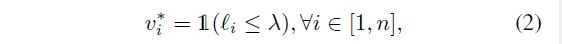# 3. Learning Curriculum from Data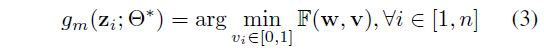## 3.1. Learning Curriculum

MentorNet可以通过1）近似存在的课程或2）发现新的课程来习得。

### Learning to approximate predefined curriculums.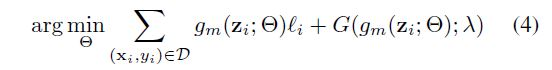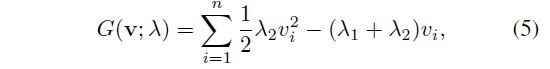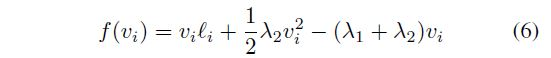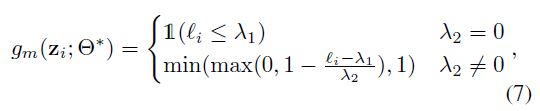### MentorNet architecture.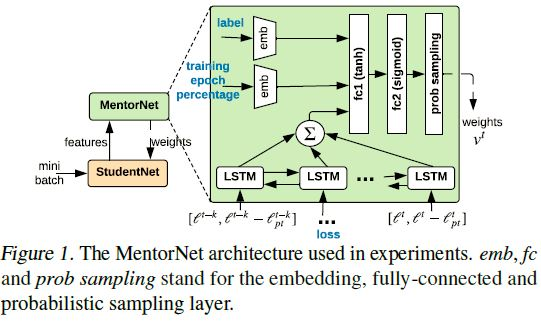## 3.2. Discussion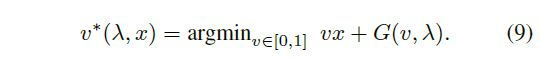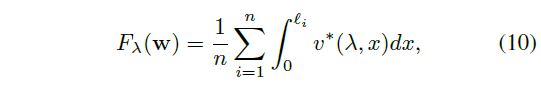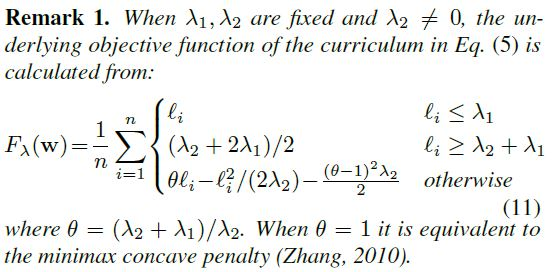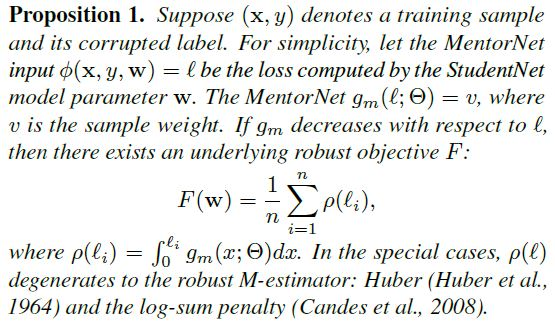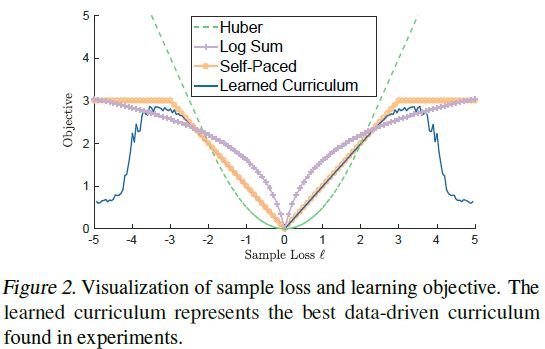# 4. The Algorithm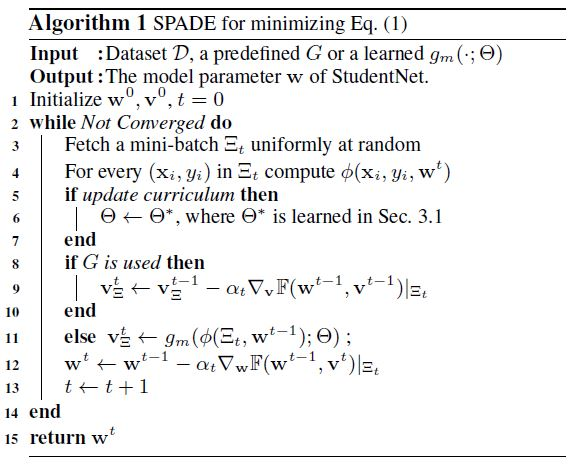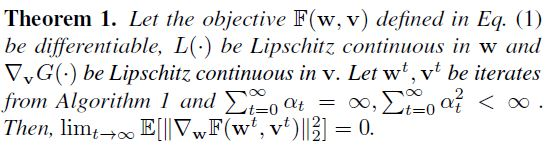# 7. Conclusions©️2019 CSDN 皮肤主题: 大白 设计师: CSDN官方博客